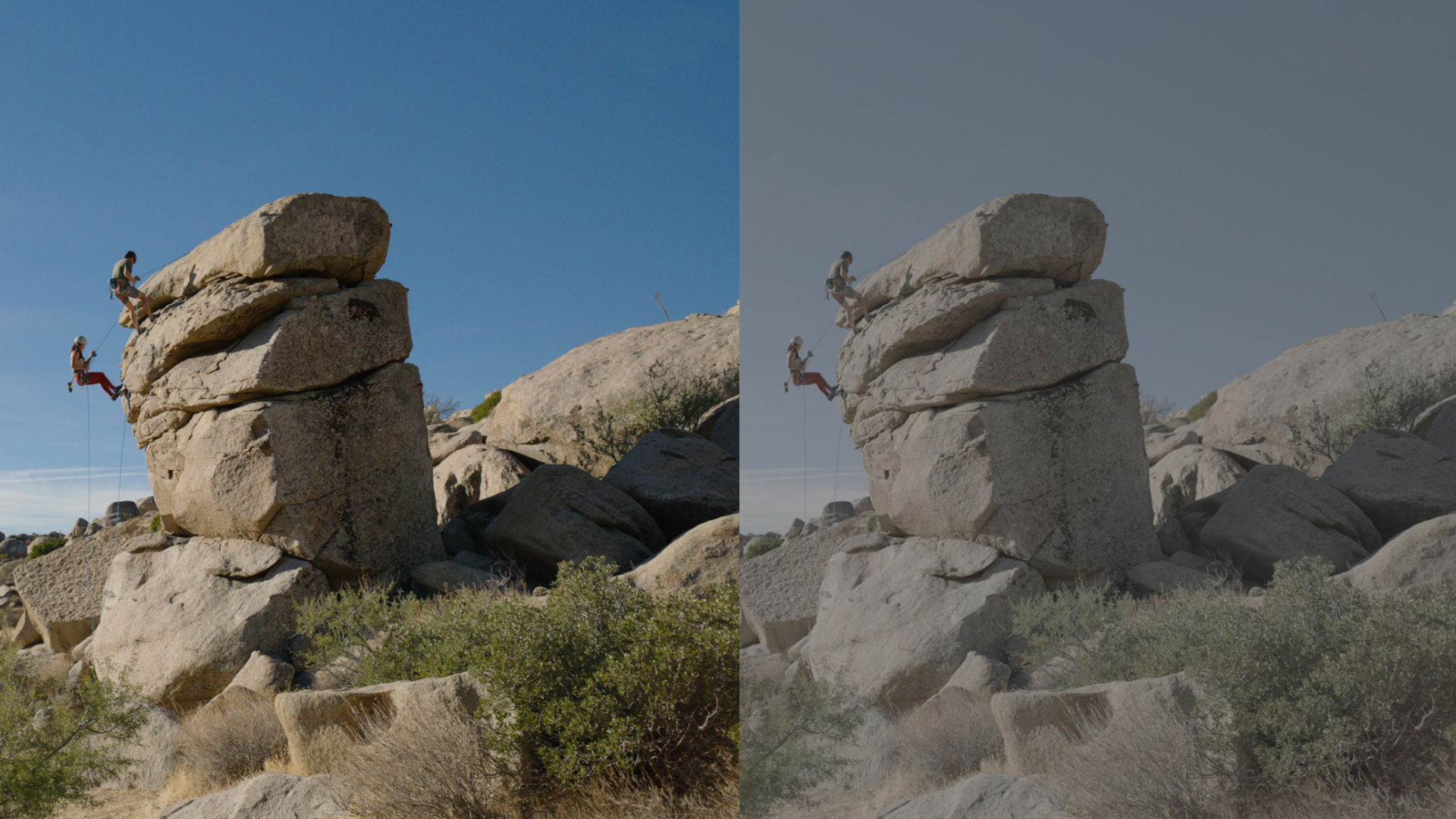# 色域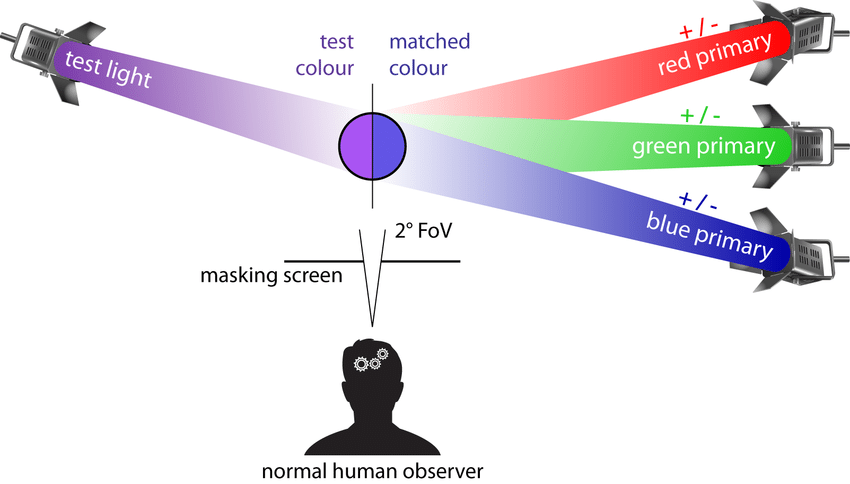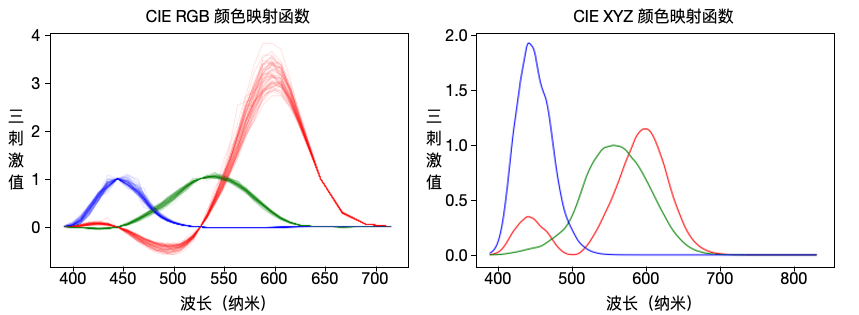\begin{aligned} x &= \dfrac{X}{X + Y + Z} \\ y &= \dfrac{Y}{X + Y + Z} \\ z &= \dfrac{Z}{X + Y + Z} = 1 - x - y \end{aligned}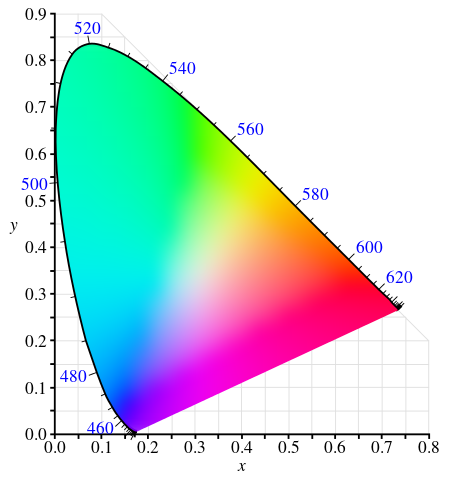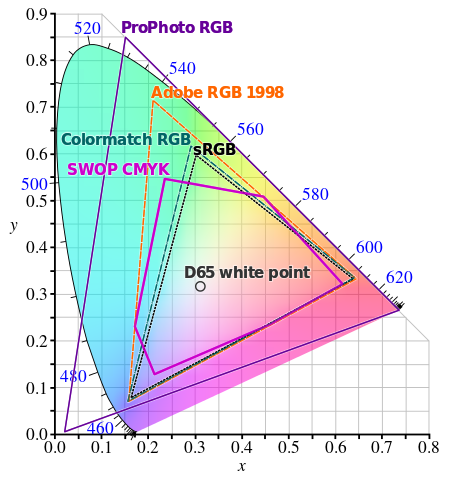# 色彩深度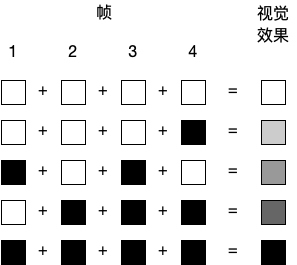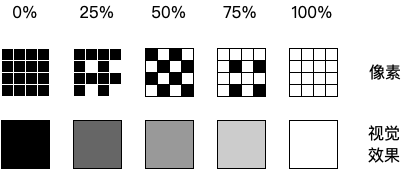# 色度抽样

• $J$ 为水平抽样的宽度
• $a$ 为第一行 $J$ 个像素中色度的抽样数量
• $b$ 为第二行 $J$ 个像素中色度的抽样数量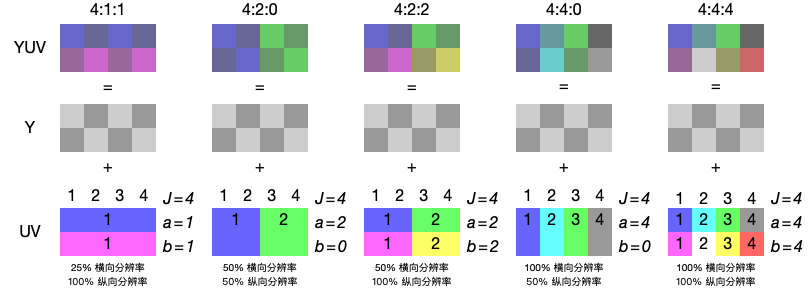# 动态范围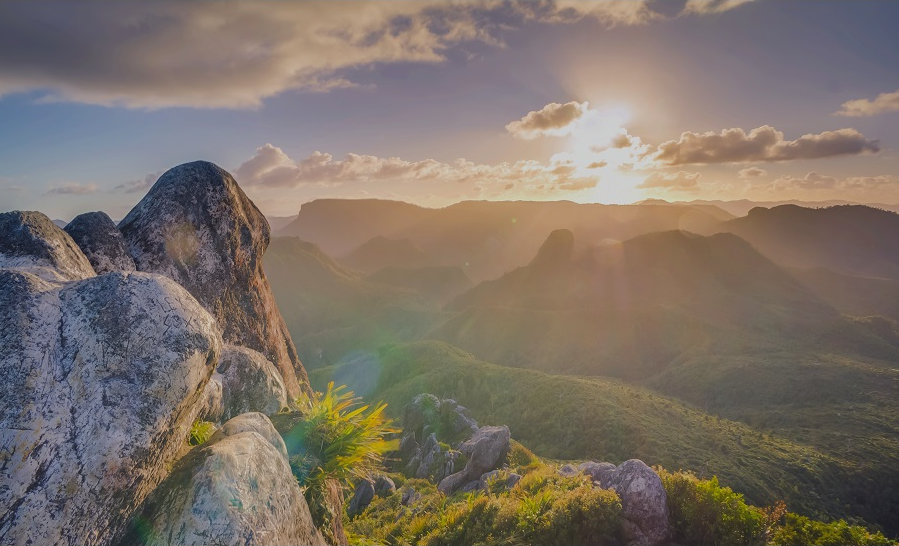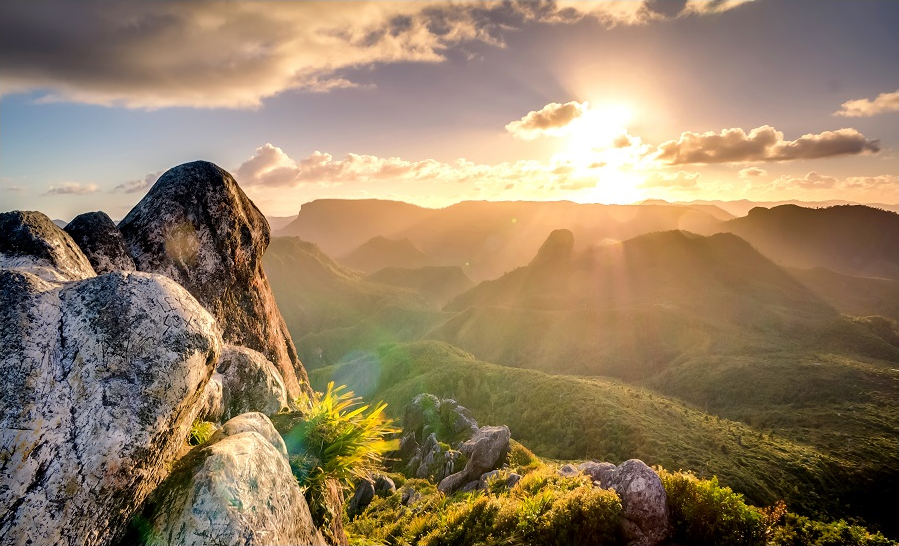1. 针对 RAW 格式照片，其存储的不同明暗数据已经足够多，针对高光降低一些曝光，暗部增加一些曝光即可获得 HDR 照片。
2. 前期进行包围曝光，即在拍摄时同时拍摄多张具有不同曝光补偿的照片，后期再利用曝光合成技术得到一张 HDR 照片。

$$H = Et$$

$$H \propto \dfrac{t}{N^2}$$

$$EV = \log_2{\dfrac{N^2}{t}} = 2 \log_2{\left(N\right)} - \log_2{\left(t\right)}$$EV 和曝光量 $\log$ 值关系